# Manuals/calci/Examples1

Engineering Examples in z3

## DESCRIPTION

• Basic Engineering examples in z3.
• Reflecting different domains like Engineering, Statistics, Medicine, etc.
• Set of cases that are progressively complex on units are used to show the user how it goes from simple to complex cases.
• Testing how we can make better solutions to the standard problems compared to other software, due to the presence of units.

## Examples

ExampleS1: Chemical Engineering
An exhaust pipe is 75mm diameter and it is cooled by surrounding it with a water jacket. The exhaust gas enters at 350C and the water enters at 10C. The surface heat transfer coefficients for the gas and water are 300 and 1500 W/m2K respectively. The wall is thin so the temperature drop due to conduction is negligible. The gasses have a mean specific heat capacity Cp of 1130 J/kgK and they must be cooled to 100C. The specific heat capacity of the water is 4190 J/kgK. The flow rate of the gas and water is 200 and 1400 kg/h respectively. Calculate the required length of pipe for parellel flow and contra flow.

```* The following example demonstrates how a problem is solved when units are used.
* The units may be SI, Imperial or a mix of both.
* Code shows how to assign a unit or convert a unit.
* 3 types of calculations are shown: Normal, using Function, using Function1 in Function2.
```
z3 code: Normal Calculation without using Function
```/*Overall Heat transfer coefficient
U = 1/((1/hg)+(1/hw)+(x/k))
//wall very thin. So, x ≈ 0
U = 1</>((1</>hg)+(1</>hw))*/

x = 0  //wall very thin
hg = 300(W/m2.degK)    //heat transfer coefficient of water
hw = 1500(W/m2.degK)   //heat transfer coefficient of gas
cpg = 1130(J/kg.degK)  //mean specific heat capacity of gas (Cp)
cpw = 4190(J/kg.degK)  //specific heat capacity of gas
mg = 200(kg/hr)        //flow rate of gas
mw = 1400(kg/hr)       //flow rate of water
D = 75mm               //given exhaust pipe diameter

tg1 = 350degC<>degK    //temp. of gas entering
tg2 = 100degC<>degK    //temp. of gas to be cooled to
tw1 = 10degC<>degK     //temp. of water entering

U = 1</>((1</>hg)<+>(1</>hw))
delt = tg1<->tg2
φ = mg<*>cpg<*>delt
tw2 = tw1<+>(φ</>(mw<*>cpw))

//Parallel flow
delti = tg1<->tw1
delt0 = tg2<->tw2
A = φ<*>(log(delt0</>delti))</>(U<*>(delt0<->delti))
L = A</>(π<*>D)

//Contra Flow
delti = tg1<->tw2
delt0 = tg2<->tw1
A = φ<*>(log(delt0</>delti))</>(U<*>(delt0<->delti))
L = A</>(π<*>D)
```

z3 code: Using Function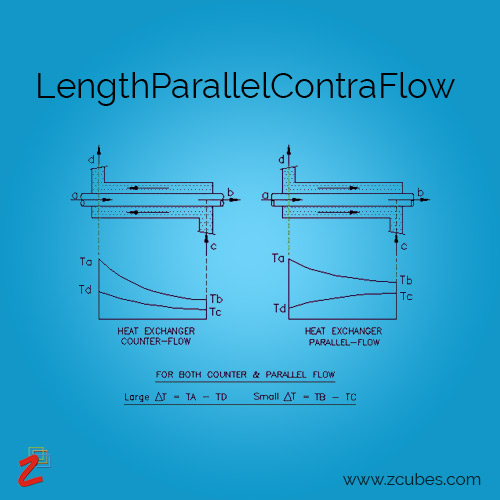```function LengthParallelContraFlow(hg,hw,cpg,cpw,mg,mw,tg1,tg2,tw1,D)
{

//Overall Heat transfer coefficient
//U = 1/((1/hg)+(1/hw)+(x/k))
var x = 0  //wall very thin
//U = 1</>((1</>hg)+(1</>hw))

var U = 1</>((1</>hg)<+>(1</>hw))
var delt = tg1<->tg2
var φ = mg<*>cpg<*>delt
var tw2 = tw1<+>(φ</>(mw<*>cpw))

//Parallel flow
var delti = tg1<->tw1
var delt0 = tg2<->tw2
var A1 = φ<*>(log(delt0</>delti))</>(U<*>(delt0<->delti))
var LParallel = A1</>(π<*>D)

//Contra Flow
delti = tg1<->tw2
delt0 = tg2<->tw1
var A2 = φ<*>(log(delt0</>delti))</>(U<*>(delt0<->delti))
var LContra = A2</>(π<*>D)

return ["LParallel=" LParallel<>m;"LContra=" LContra<>m]
}

hg = 300(W/m2.degK)     //heat transfer coefficient of water
hw = 1500(W/m2.degK)    //heat transfer coefficient of gas
cpg = 1130(J/kg.degK)   //mean specific heat capacity of gas (Cp)
cpw = 4190(J/kg.degK)   //specific heat capacity of gas
mg = 200(kg/hr)         //flow rate of gas
mw = 1400(kg/hr)        //flow rate of water
D = 75mm                //given exhaust pipe diameter
tg1 = 350degC<>degK     //temp. of gas entering
tg2 = 100degC<>degK     //temp. of gas to be cooled to
tw1 = 10degC<>degK      //temp. of water entering

LengthParallelContraFlow(hg,hw,cpg,cpw,mg,mw,tg1,tg2,tw1,D)
```

Result:

```LParallel=	1.4801324499356323m
LContra=	1.4414317039664961m
```

z3 code: Multi-calculation Using Function(variable diameter)```function LengthParallelContraFlow(hg,hw,cpg,cpw,mg,mw,tg1,tg2,tw1,D)
{

//Overall Heat transfer coefficient
//U = 1/((1/hg)+(1/hw)+(x/k))
var x = 0  //wall very thin
//U = 1</>((1</>hg)+(1</>hw))

var U = 1</>((1</>hg)<+>(1</>hw))
var delt = tg1<->tg2
var φ = mg<*>cpg<*>delt
var tw2 = tw1<+>(φ</>(mw<*>cpw))

//Parallel flow
var delti = tg1<->tw1
var delt0 = tg2<->tw2
var A1 = φ<*>(log(delt0</>delti))</>(U<*>(delt0<->delti))
var LParallel = A1</>(π<*>D)

//Contra Flow
delti = tg1<->tw2
delt0 = tg2<->tw1
var A2 = φ<*>(log(delt0</>delti))</>(U<*>(delt0<->delti))
var LContra = A2</>(π<*>D)

return (LParallel<>m)
}

LengthParallelContraFlow ⩨;

hg = 300(W/m2.degK)     //heat transfer coefficient of water
hw = 1500(W/m2.degK)    //heat transfer coefficient of gas
cpg = 1130(J/kg.degK)   //mean specific heat capacity of gas (Cp)
cpw = 4190(J/kg.degK)   //specific heat capacity of gas
mg = 200(kg/hr)         //flow rate of gas
mw = 1400(kg/hr)        //flow rate of water<>
D = (75..80)<>mm        /*given exhaust pipe diameter*/
tg1 = 350degC<>degK     //temp. of gas entering
tg2 = 100degC<>degK     //temp. of gas to be cooled to
tw1 = 10degC<>degK      //temp. of water entering

LengthParallelContraFlow(hg,hw,cpg,cpw,mg,mw,tg1,tg2,tw1,D)
```

Result:

```      hg              hw             cpg              cpw           mg               mw            tg1    tg2      tw1   D            LParallel
300W.m-2.K-1	1500W.m-2.K-1	1130J.kg-1.K-1	4190J.kg-1.K-1	200kg.hr-1	1400kg.hr-1	623.15K	373.15K	283.15K	75mm	1.4801324499356323m
300W.m-2.K-1	1500W.m-2.K-1	1130J.kg-1.K-1	4190J.kg-1.K-1	200kg.hr-1	1400kg.hr-1	623.15K	373.15K	283.15K	76mm	1.460657022962795m
300W.m-2.K-1	1500W.m-2.K-1	1130J.kg-1.K-1	4190J.kg-1.K-1	200kg.hr-1	1400kg.hr-1	623.15K	373.15K	283.15K	77mm	1.4416874512360054m
300W.m-2.K-1	1500W.m-2.K-1	1130J.kg-1.K-1	4190J.kg-1.K-1	200kg.hr-1	1400kg.hr-1	623.15K	373.15K	283.15K	78mm	1.4232042787842618m
300W.m-2.K-1	1500W.m-2.K-1	1130J.kg-1.K-1	4190J.kg-1.K-1	200kg.hr-1	1400kg.hr-1	623.15K	373.15K	283.15K	79mm	1.405189034749018m
300W.m-2.K-1	1500W.m-2.K-1	1130J.kg-1.K-1	4190J.kg-1.K-1	200kg.hr-1	1400kg.hr-1	623.15K	373.15K	283.15K	80mm	1.3876241718146551m
```

z3 code: USING EXAMPLE1 function SOLUTION IN EXAMPLE2 function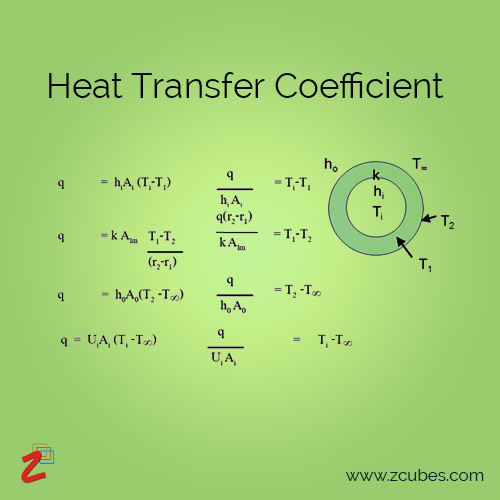```function HeatTransferCoefficient(hg,hw)
{
//Overall Heat transfer coefficient
//U = 1/((1/hg)+(1/hw)+(x/k))
var x = 0  //wall very thin
//U = 1</>((1</>hg)+(1</>hw))

var U = 1</>((1</>hg)<+>(1</>hw))

return [U]
}

hg = 300(W/m2.degK)
hw = 1500(W/m2.degK)

HeatTransferCoefficient(hg,hw)
/*250W.m-2 .K-1*/

function LengthParallelContraFlow(tw1,mg,mw,cpg,cpw,tg1,tg2)
{

var delt = tg1<->tg2
var φ = mg<*>cpg<*>delt
var tw2 = tw1<+>(φ</>(mw<*>cpw))

//Parallel flow
var delti = tg1<->tw1
var delt0 = tg2<->tw2
var A1 = φ<*>(log(delt0</>delti))</>(HeatTransferCoefficient(hg,hw)<*>(delt0<->delti))
var LParallel = A1</>(π<*>D)

//Contra Flow
delti = tg1<->tw2
delt0 = tg2<->tw1
var A2 = φ<*>(log(delt0</>delti))</>(HeatTransferCoefficient(hg,hw)<*>(delt0<->delti))
var LContra = A2</>(π<*>D)

return ["LParallel=" LParallel<>m;"LContra=" LContra<>m]
}

cpg = 1130(J/kg.degK)
cpw = 4190(J/kg.degK)
mg = 200(kg/hr)
mw = 1400(kg/hr)
tw1 = 10degC<>degK
tg1 = 350degC<>degK
tg2 = 100degC<>degK

LengthParallelContraFlow(tw1,mg,mw,cpg,cpw,tg1,tg2)
```

Result:

```LParallel=	1.4801324499356323m
LContra=	1.4414317039664961m
```

ExampleS2: Civil Engineering
A steel pipe 5 ft (1.5 m) in diameter and 3/5 in. (9.53 mm) thick sustains a fluid pressure of 180 lb/sq.in. (1241.1 kPa). Determine the hoop stress, the longitudinal stress, and the increase in diameter of this pipe. Use 0.25 for Poisson’s ratio.

z3 code: Using Function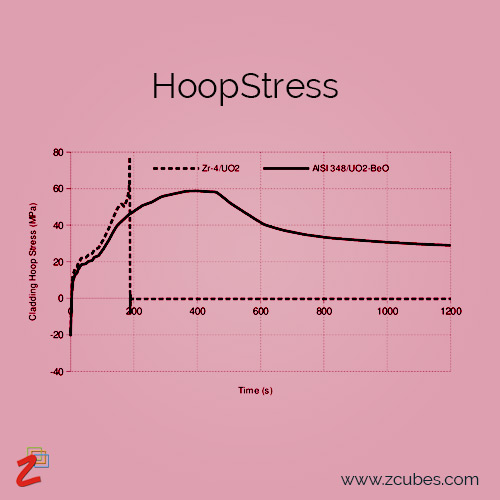```function HoopStress(p,D,t,E,v){

/*hoop stress
s = pD/2t
longitudinal stress
s'= pD/4t
increase in cyl diameter
delD = D(s-vs')/E */

var s = p<*>D</>(2<*>t)
var sdash = p<*>D</>(4<*>t)
var delD = D<*>(s<->v<*>sdash)</>E

return ["s=" s;"sdash=" sdash;"delD=" delD<>inch]
}

p = 180(lb/sqin)   // radial pressure
D = 5(ft)          // internal diameter
t = (3/8)<>(inch)  // wall thickness
E = 30e+6(lb/sqin) // modulus of elasticity for steel
v = 0.25  //poison's ratio

HoopStress(p,D,t,E,v)
```

Result:

```s =	14400lb.sqin-1
sdash =	7200lb.sqin-1
delD =	0.0252inch
```

ExampleS3: Civil Engineering
A 1/2-in. (12.7-mm) diameter Copperweld bar consists of a steel core 3/8 in. (9.53 mm) indiameter and a copper skin 1/16 in. (1.6 mm) thick. What is the elongation of a 1-ft (0.3-m) length of this bar, and what is the internal force between the steel and copper arising from a temperature rise of 80°F (44.4°C)? Use the following values for thermal expansion coefficients: cs = 6.5*106 and cc = 9.0*106 , where the subscripts s and c refer to steel and copper, respectively. Also, Ec = 15*106 lb/sq.in. (1.03*108 kPa).

z3 code: Normal Calculation without using Function
```dc = 12.7(mm)         // diameter of copperweld
ds = (3/8)<>(inch)    // diameter of steel core
dcs = (1/16)<>(inch)  // diameter of copper skin
Es = 30e+6(lbf/in2)   // modulus of elasticity for steel
Ec = 1.03e+11(Pa)     // modulus of elasticity for copper
cs = 6.5e-6(diffF-1)  // coefficient of thermal expansion steel
cc = 9e-6(diffF-1)    // coefficient of thermal expansion copper
L = 1(ft)             // original length
delT = 44.4(diffC)    // increase in temperature

//cross-sectional area
A = π<*>(dc)^2</>4
As = π<*>(ds)^2</>4
Ac = A<->As

//coeff of expansion
//c = ((As<*>Es<*>cs)<+>(Ac<*>Ec<*>cc))</>(As<*>Es<+>Ac<*>Ec)
a = (As<*>Es<*>cs)
b = (Ac<*>Ec<*>cc)<>(lbf.diffF-1)
d = (As<*>Es<+>Ac<*>Ec)
c = (a<+>b)</>d

//thermal expansion
delL = c<*>L<*>delT

//expansion w.o restraint
delLc = cc<*>L<*>delT
delLcs = delLc<->delL
delLs= cs<*>L<*>delT
delLsc = delL<->delLs

//restraining force
P1 = Ac<*>Ec<*>delLcs</>L
P2 = As<*>Es<*>delLsc</>L
```

z3 code: Using Function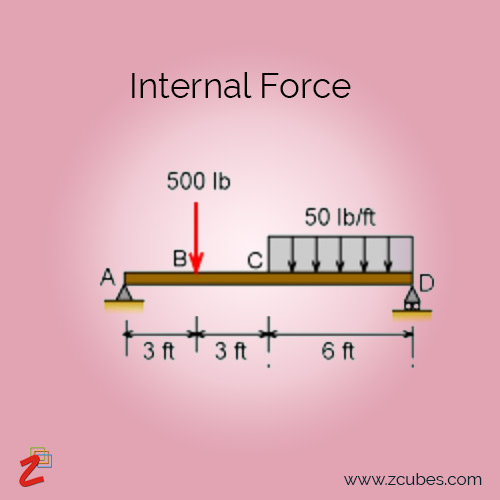```function InternalForce(dc,ds,dcs,L,Es,Ec,cs,cc,delT){

//cross-sectional area
var A = π<*>(dc)^2</>4
var As = π<*>(ds)^2</>4
var Ac = A<->As

//coeff of expansion
//c = ((As<*>Es<*>cs)<+>(Ac<*>Ec<*>cc))</>(As<*>Es<+>Ac<*>Ec)
var a = (As<*>Es<*>cs)
var b = (Ac<*>Ec<*>cc)<>(lbf.diffF-1)
var d = (As<*>Es<+>Ac<*>Ec)
var c = (a<+>b)</>d

//thermal expansion
var delL = c<*>L<*>delT

//expansion w.o restraint
var delLc = cc<*>L<*>delT
var delLcs = delLc<->delL
var delLs= cs<*>L<*>delT
var delLsc = delL<->delLs

//restraining force
var P1 = Ac<*>Ec<*>delLcs</>L
var P2 = As<*>Es<*>delLsc</>L

return ["P1=" P1; "P2 =" P2]
}

dc = 12.7(mm)         // diameter of copperweld
ds = (3/8)<>(inch)    // diameter of steel core
dcs = (1/16)<>(inch)  // diameter of copper skin
Es = 30e+6(lbf/in2)   // modulus of elasticity for steel
Ec = 1.03e+11(Pa)     // modulus of elasticity for copper
cs = 6.5e-6(diffF-1)  // coefficient of thermal expansion steel
cc = 9e-6(diffF-1)    // coefficient of thermal expansion copper
L = 1(ft)             // original length
delT = 44.4(diffC)    // increase in temperature

InternalForce(dc,ds,dcs,L,Es,Ec,cs,cc,delT)
```

Result:

```P1=	184.81887968319361lbf
P2 =	184.81901805992197lbf
```

z3 code: USING EXAMPLE1 function SOLUTION IN EXAMPLE2 function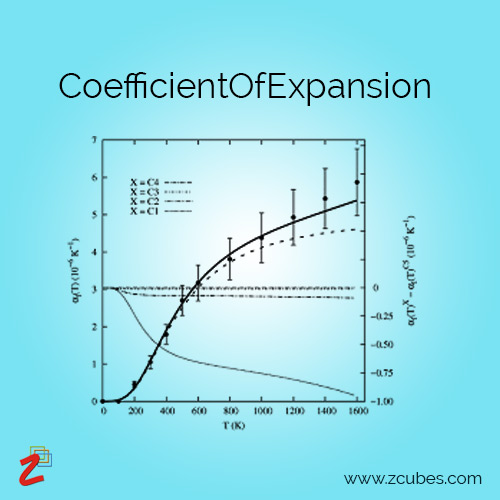```function CoefficientOfExpansion(dc,ds,dcs,Es,Ec,cs,cc){

//cross-sectional area
var A = π<*>(dc)^2</>4
var As = π<*>(ds)^2</>4
var Ac = A<->As

//coeff of expansion
//c = ((As<*>Es<*>cs)<+>(Ac<*>Ec<*>cc))</>(As<*>Es<+>Ac<*>Ec)
var a = (As<*>Es<*>cs)
var b = (Ac<*>Ec<*>cc)<>(lbf.diffF-1)
var d = (As<*>Es<+>Ac<*>Ec)
var c = (a<+>b)</>d

return[As,Ac,c]
}

dc = 12.7(mm)
ds = (3/8)<>(inch)
dcs = (1/16)<>(inch)
Es = 30e+6(lbf/in2)
Ec = 1.03e+11(Pa)
cs = 6.5e-6(diffF-1)
cc = 9e-6(diffF-1)

CoefficientOfExpansion(dc,ds,dcs,Es,Ec,cs,cc)

/*As=	0.11044661672776616inch2
Ac=	0.0859029241215959inch2
c=	0.000007197944655660324diffF-1*/

function InternalForce(L,delT,Es,Ec,cs,cc){

//thermal expansion
var delL = CoefficientOfExpansion(dc,ds,dcs,Es,Ec,cs,cc)<*>L<*>delT

//expansion w.o restraint
var delLc = cc<*>L<*>delT
var delLcs = delLc<->delL
var delLs= cs<*>L<*>delT
var delLsc = delL<->delLs

//restraining force
var P1 = CoefficientOfExpansion(dc,ds,dcs,Es,Ec,cs,cc)<*>Ec<*>delLcs</>L
var P2 = CoefficientOfExpansion(dc,ds,dcs,Es,Ec,cs,cc)<*>Es<*>delLsc</>L

return ["P1=" P1;"P2=" P2]
}

L = 1(ft)
delT = 44.4(diffC)
Es = 30e+6(lbf/in2)
Ec = 1.03e+11(Pa)
cs = 6.5e-6(diffF-1)
cc = 9e-6(diffF-1)

InternalForce(L,delT,Es,Ec,cs,cc)
```

Result:

```P1=	184.81887968319361lbf
P2=	184.81901805992197lbf
```

ExampleS4: Civil Engineering
M1 is a 4x4, F = 5500 lb (24,464 N), and Phi = 30°. The allowable compressive stresses are P = 1200 lb/sq.in. (8274 kPa) and Q = 390 lb/sq.in. (2689.1 kPa). The projection of M1 into M2 is restricted to a vertical distance of 2.5 in. (63.5 mm).

z3 code: Using Function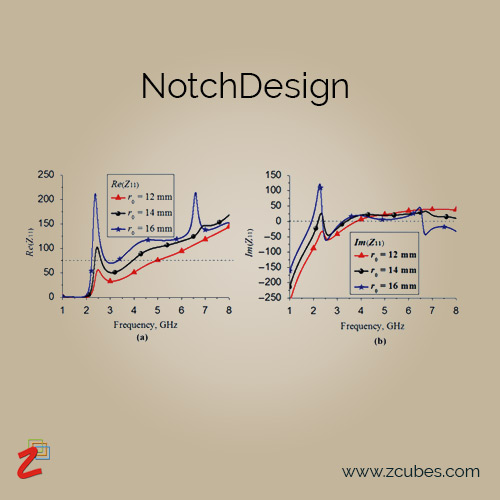```function NotchDesign(b,φ,P,Q,F,A){

//lengths
var AB = b</>DSIN(φ)
var AC = (b<*>DSIN(φ/2))</>DSIN(φ)
var BC = (b<*>DCOS(φ/2))</>DSIN(φ)

//stresses f1 and f2
var f1 = (F<*>DSIN(φ))</>(A<*>DTAN(φ/2))
var f2 = (F<*>DSIN(φ)<*>DTAN(φ/2))</>(A)

//allowable stresses
var N1 = P<*>Q</>((P<*>(DSIN(φ/2))^2)<+>Q<*>(DCOS(φ/2))^2)
var N2 = P<*>Q</>((P<*>(DCOS(φ/2))^2)<+>Q<*>(DSIN(φ/2))^2)

return["AC=" AC;"BC=" BC;"f1=" f1<>(lbf/sqin);"f2=" f2<>(lbf/sqin);"N1=" N1<>Pa;"N2=" N2<>Pa]
}

b = 3.625(inch)     // length
φ = 30(deg)         // angle
P = 1200(lbf/sqin)  // allowable compressive stress
Q = 2689.1e+3(Pa)   // allowable compressive stress
F = 24464(N)        // force
A = 13.1(sqin)      // area

NotchDesign(b,φ,P,Q,F,A)
```

Result:

```AC=	1.8764380769932756inch
BC=	7.002962240595746inch
f1=	783.4067480901655lbf.sqin-1
f2=	56.2460738932418lbf.sqin-1
N1=	7263269.6857909495Pa
N2=	2816446.5788098425Pa
```

ExampleS5: Engineering Economics
The QRS Corp. purchased capital equipment for use in a 5-year venture. The equipment cost \$240,000 and had zero salvage value. If the income tax rate was 52 percent and the annual income from the investment was \$83,000 before taxes and depreciation, what was the average rate of earnings if the profits after taxes were invested in tax-free bonds yielding 3 percent? Compare the results obtained when depreciation is computed by the straight-line method.

z3 code: Using Function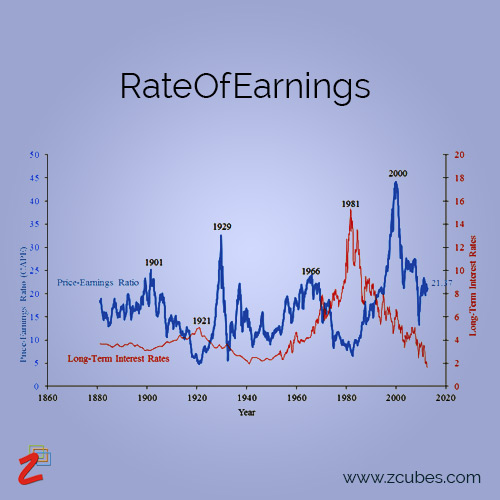```function RateOfEarnings(EC,n,GI,r,i){

//taxable income
var DC = EC</>n
var TI = GI<->DC

//annual tax payment
var TP = r<*>TI

//net income
var NI = GI<->TP
//S = R(USCA)
//SPCA = (1<+>i)^n
var s = NI<*>(5.309)
var sp = s</>EC
var m = [(sp^0.2)<->1]<*>100

return m
}

EC = 240000(USD) // equipment cost
n = 5       // no. of years
GI = 83000  // gross income
r = 0.52    // income tax rate
i = 0.03    // tax free bonds yeild

RateOfEarnings(EC,n,GI,r,i)
```

Result:

```m= 7.467042666032353
```

ExampleS6: Fluid Mechanics
A steel pipe is discharging 10 ft3/s (283.1 L/s) of water. At section 1, the pipe diameter is 12 in. (304.8 mm), the pressure is 18 lb/sq.in. (124.11 kPa), and the elevation is 140 ft(42.67 m). At section 2, farther downstream, the pipe diameter is 8 in. (203.2 mm), and the elevation is 106 ft (32.31 m). If there is a head loss of 9 ft (2.74 m) between these sections due to pipe friction, what is the pressure at section 2?

z3 code: Using Function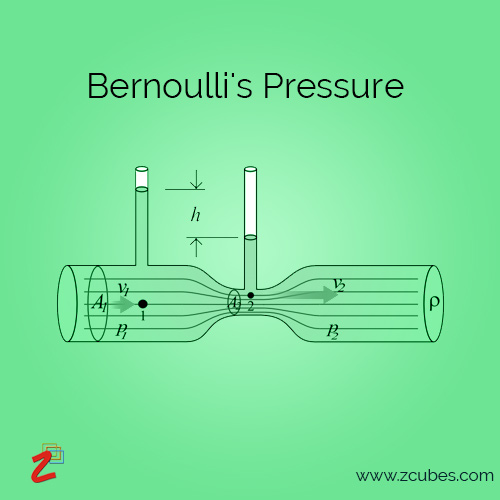```function BernoullisPressure(d1,d2,p1,z1,z2,q1,q2,hf,w,g){

var a1 = π<*>(d1)^2</>4
var a2 = π<*>(d2)^2</>4
var v1 = q1</>a1
var v2 = q2</>a2

var p2 = (((v1^2<->v2^2)</>(2<*>g)<+>z1<->z2<->hf)<*>w)<+>p1

return p2<>(lbf/in2)
}

d1 = 12(inch)       // pipe1 diameter
d2 = 203.2(mm)      // pipe2 diameter
p1 = 124.11e+3(Pa)  // pressure at 1
z1 = 140(ft)        // height at 1
z2 = 32.31(m)       // height at 2
q1 = 283.1(L/s)     // water discharge at 1
q2 = 10(ft3/s)      // water discharge at 2
hf = 9(ft)          // head loss
w = (62.4/(144*12))<>(lbf/inch3)  // specific weight
g = 32.2(ft/s2)     // gravitational force

BernoullisPressure(d1,d2,p1,z1,z2,q1,q2,hf,w,g)
```

Result:

```p2= 24.400241276116066lbf.in-2
```

z3 code: Multi-calculation Using Function(variable diameter)```function BernoullisPressure(d1,d2,p1,z1,z2,q1,q2,hf,w,g){

var a1 = π<*>(d1)^2</>4
var a2 = π<*>(d2)^2</>4
var v1 = q1</>a1
var v2 = q2</>a2

var p2 = (((v1^2<->v2^2)</>(2<*>g)<+>z1<->z2<->hf)<*>w)<+>p1

return p2<>(lbf/in2)
}

BernoullisPressure ⩨;

d1 = (1..5)<>(inch)       // pipe1 diameter
d2 = 203.2(mm)      // pipe2 diameter
p1 = 124.11e+3(Pa)  // pressure at 1
z1 = 140(ft)        // height at 1
z2 = 32.31(m)       // height at 2
q1 = 283.1(L/s)     // water discharge at 1
q2 = 90<>(ft3/s)      // water discharge at 2
hf = 9(ft)          // head loss
w = (62.4/(144*12))<>(lbf/inch3)  // specific weight
g = 32.2(ft/s2)     // gravitational force

BernoullisPressure(d1,d2,p1,z1,z2,q1,q2,hf,w,g)
```

Result:

```d1         d2      p1             z1      z2        q1             q2            hf                  w                       g                    p2
1inch	203.2mm	124110Pa	140ft	32.31m	283.1L.s-1	90ft3.s-1	9ft	0.03611111111111111lbf.inch-3	32.2ft.s-2	22190.006973772463lbf.in-2
2inch	203.2mm	124110Pa	140ft	32.31m	283.1L.s-1	90ft3.s-1	9ft	0.03611111111111111lbf.inch-3	32.2ft.s-2	994.5545670303857lbf.in-2
3inch	203.2mm	124110Pa	140ft	32.31m	283.1L.s-1	90ft3.s-1	9ft	0.03611111111111111lbf.inch-3	32.2ft.s-2	-139.3585246883264lbf.in-2
4inch	203.2mm	124110Pa	140ft	32.31m	283.1L.s-1	90ft3.s-1	9ft	0.03611111111111111lbf.inch-3	32.2ft.s-2	-330.16120839099426lbf.in-2
5inch	203.2mm	124110Pa	140ft	32.31m	283.1L.s-1	90ft3.s-1	9ft	0.03611111111111111lbf.inch-3	32.2ft.s-2	-382.30202131157984lbf.in-2
```

## Unit Conversion Examples

ExampleP1:
An oil with density(ρ) of 999.834907696(kg/m3) flows through a pipe of length(L) 30.48m and diameter(D) 0.1524m with a nominal velocity(U) of 0.94488(m/s). Pressure drop(dP) in the pipe is 1723.68925(Pa). Find the friction coeffecient.

z3 code: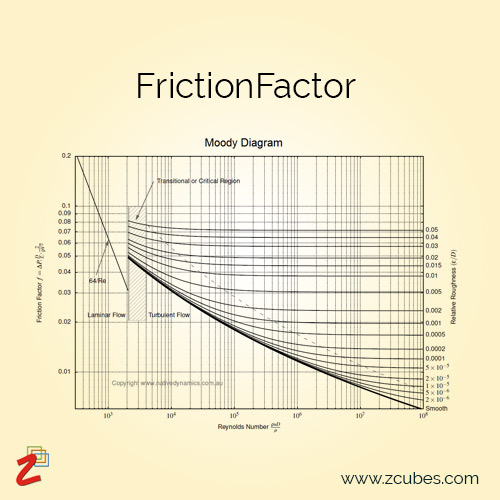```/*solution with SI units*/
FrictionFactor=(dP,L,D,ρ,U)=>[dP,L,D,ρ,U,(dP</>((L</>D)<*>(0.5<*>ρ<*>(U<^>2))))];
dP = 1723.68925(Pa)        // pressure drop
L = 30.48m                 // pipe length
D = 0.1524m                // pipe diameter
ρ = 999.834907696(kg/m3)   // density
U = 0.94488(m/s)           // nominal velocity
FrictionFactor(dP,L,D,ρ,U)
//0.019309781729077106

/*solution with SI and Imperial units*/
FrictionFactor=(dP,L,D,ρ,U)=>[dP,L,D,ρ,U,(dP</>((L</>D)<*>(0.5<*>ρ<*>(U<^>2))))];
dP = 1723.68925(Pa)
L = 100ft
D = 0.1524m
ρ = 1.94(slug/ft3)
U = 0.94488(m/s)
FrictionFactor(dP,L,D,ρ,U)
//0.019309781729077106

/*solution with imperial units*/
FrictionFactor=(dP,L,D,ρ,U)=>[dP,L,D,ρ,U,(dP</>((L</>D)<*>(0.5<*>ρ<*>U^2)))];
// note the arrow notation function here. Helps with shading the variables from global scope etc. compared to FrictionFactor:=[dP,L,D,ρ,U,(dP</>((L</>D)<*>(0.5<*>ρ<*>U^2)))] notation.
dP = 0.25(psi)
L = 100ft
D = 6inch
ρ = 1.94(slug/ft3)
U = 3.1(ft/s)
FrictionFactor(dP,L,D,ρ,U)
//0.019309781729077106
```

ExampleP2:
Find the Reynolds number if a fluid of viscosity 0.4 N/m2 and relative density of 900 Kg/m3 through a 20 mm pipe with a Velocity of 2.5 m/s?

z3 code:
z3 code: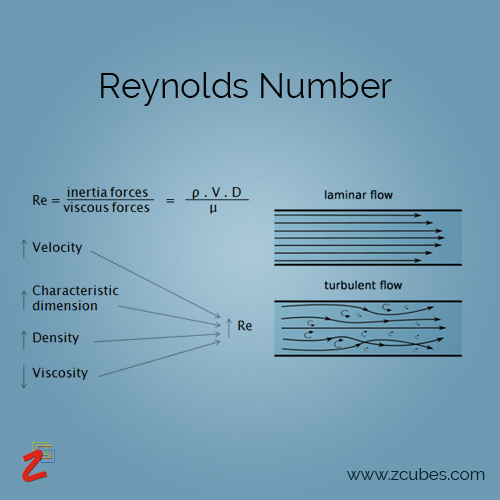```/*solution in S.I. units*/
ReynoldsNumber:=[v,d,rho,mu,v<*>d<*>rho</>mu];
rho = 900(kg/m3)              // density
v = 2.5(m/s)                  // velocity
mu = 0.4(N.s/m2)              // viscosity
d = 20(mm)                    // diameter
ReynoldsNumber(v,d,rho,mu)
//112.5

/*solution with SI and Imperial units*/
ReynoldsNumber:=[v,d,rho,mu,v<*>d<*>rho</>mu];
rho = 56.185(lb/ft3)
v = 2.5(m/s)
mu = 0.4(N.s/m2)<>(cP)
d = 2(cm)
rn=ReynoldsNumber(v,d,rho,mu);
rn<>_ ; // _ is used as an indicator to reduce the final units to minimal units, in this case unitless.
//112.5

/*solution in imperial units*/
ReynoldsNumber:=[v,d,rho,mu,v<*>d<*>rho</>mu];
rho = 56.185(lb/ft3)
v = 8.20209(ft/s)
mu = 0.00835(lbf.s/ft2)
d = 0.0656(ft)
ReynoldsNumber(v,d,rho,mu)
//112.5
```

ExampleP3:
A slab that is made from copper has a length of 10 cm and an area that is 90 cm2. The front side is heated to 150 oC and the back to 10 oC. Find the heat flux q and the heat flow rate Q in the slab once steady state is reached. Assume dT/dx is constant.

z3 code: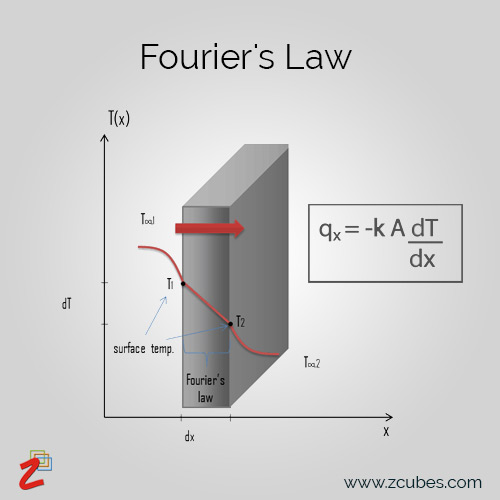```/*solution in S.I units*/
FouriersLaw=(k,a,delt,delx)=>[k,a,delt,delx,(-1)<*>(k)<*>a<*>delt</>delx];
k = 401("W.m-1.diffK-1")         //
a = 90(cm2)                    // area
t1 = 10(degC)<>(degK)          // temperature1
t2 = 150(degC)<>(degK)         // temperature2
delt = t1<->t2                 // diff.of temperature
delx = 0.1(m)                  // diff.in length
FouriersLaw(k,a,delt,delx)
//5052.59999W.diffK-1 .K
//In the solution above diffK and K can be cancelled out.
//Solution = 5052.59999W

/*solution with SI and Imperial units*/
FouriersLaw=(k,a,delt,delx)=>[k,a,delt,delx,(-1)<*>(k)<*>a<*>delt</>delx];
k = 417.0483("BTUIT*h-1*ft-1*diffK-1")
a = 90(cm2)
t1 = 10(degC)<>(degK)
t2 = 150(degC)<>(degK)
delt = t1<->t2
delx = 0.1(m)
FouriersLaw(k,a,delt,delx)
//417.0483BTUIT.h-1.ft-1.diffK-1	90cm2	-140K	0.1m	17240.18562992126BTUIT.diffK-1.h-1.K

/*solution in imperial units*/
FouriersLaw=(k,a,delt,delx)=>[k,a,delt,delx,(-1)<*>(k)<*>a<*>delt</>delx];
k = 417.0483("BTUIT*h-1*ft-1*diffK-1")
a = 0.096875(ft2)
t1 = 10(degC)<>(degK)
t2 = 150(degC)<>(degK)
delt = t1<->t2
delx = 0.32808(ft)
FouriersLaw(k,a,delt,delx)
//17240.360792337236BTUIT.diffK-1 .h-1 .K = 5052.599W
```

ExampleP4:
A body is projected with a velocity of 20 ms-1 at 50deg to the horizontal. Find Maximum height reached.

z3 code: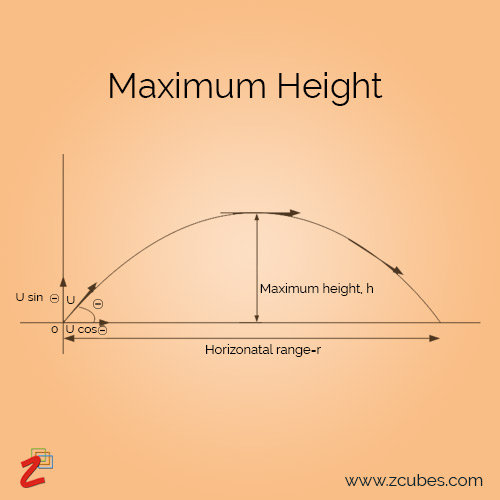```/*solution in S.I units*/
MaximumHeight:=[v0,a,g,v0<^>2<*>(DSIN(a))<^>2</>(2<*>g)];
v0 = 20(m/s)
a = 50
g = 9.8(m/s2)
MaximumHeight(v0,a,g)
//11.97600181292786m

/*solution in imperial units*/
MaximumHeight:=[v0,a,g,v0<^>2<*>(DSIN(a))<^>2</>(2<*>g)];
v0 = 65.6167(ft/s)
a = 50
g = 23149.624(ipm/s)
MaximumHeight(v0,a,g)
//0.054571107280907624ft2.ipm-1.s-1 <> 11.97600181292786m

/*solution with SI and Imperial units*/
MaximumHeight:=[v0,a,g,v0<^>2<*>(DSIN(a))<^>2</>(2<*>g)];
v0 = 20(m/s)
a = 50
g = 23149.624(ipm/s)
MaximumHeight(v0,a,g)
//0.054571107280907624ft2.ipm-1.s-1<> 11.97600181292786m
```

ExampleP5:
A sound source with a frequency of 790Hz moves away from a stationary observer at a rate of 15m/s. What frequency does the observer hear? The speed of sound is 340m/s.

z3 code:```/*solution in S.I units*/
DopplerEffect:=[v,vl,vs,fs,(v<+>vl)<*>fs</>(v<+>vs)]
v = 340(m/s)
vl = 0(m/s)
vs = 15(m/s)
fs = 790(Hz)
DopplerEffect(v,vl,vs,fs)
//756.6197183098592Hz

/*solution with SI and Imperial units*/
DopplerEffect:=[v,vl,vs,fs,(v<+>vl)<*>fs</>(v<+>vs)]
v = 1115.485(ft/s)
vl = 0(m/s)
vs = 29.1576(kn)
fs = 790(Hz)
DopplerEffect(v,vl,vs,fs)<>Hz
//389.2388081250533Hz.kn-1 .m.s-1 <> 756.6197183098592Hz

/*solution in imperial units*/
DopplerEffect:=[v,vl,vs,fs,(v<+>vl)<*>fs</>(v<+>vs)]
v = 1115.485(ft/s)
vl = 0(ft/s)
vs = 49.21248(ft/s)
DopplerEffect(v,vl,vs,fs)
//756.6197790691537Hz
```

ExampleP6:
Circular Segment Area

z3 code: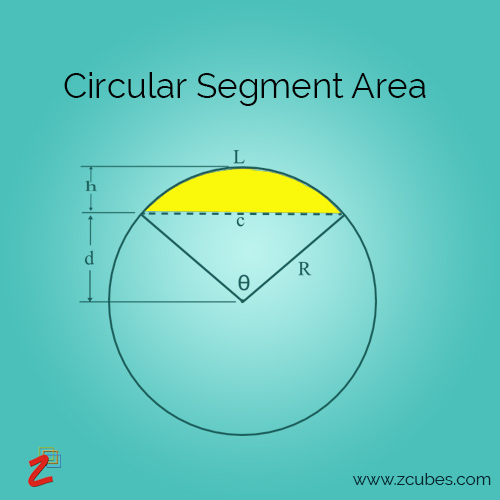```CircularSegmentArea:=[r,α,(r^2<*>(RAD(α)<->DSIN(α))</>2)];
//φ = 2<*>(ACOS((r<->2r)</>r))
//α-Angle
r = 2m
α = 30(deg)
CircularSegmentArea(r,α)
//0.04719755119659774m2.㎭

r = 2(m)
CircularSegmentArea(r,α)
//0.04719740850564924m2.㎭

r = 2(m)
CircularSegmentArea(r,α)
//0.04719755119659774m2.㎭
```

ExampleP7:
Coulombs Law

z3 code: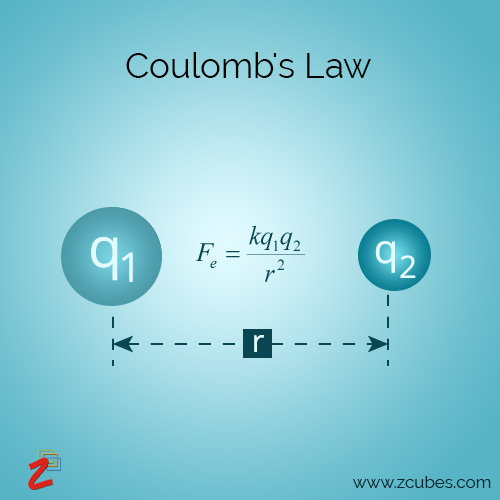```CoulombsLaw:=[q1,q2,k,r,q1<*>q2<*>k</>r<^>2];
q1 = 1e-6(C)
q2 = 1e-6(C)
r = 1(cm)
k = 8.99e9(N.m2/C2)
CoulombsLaw(q1,q2,k,r)
//89.89999999999999kg.m.s-2

CoulombsLaw:=[q1,q2,k,r,q1<*>q2<*>k</>r<^>2];
q1 = 1.0364268992774003e-11(F)
q2 = 1.0364268992774003e-11(F)
r = 1(cm)
k = 8.99e9(N.m2/C2)
CoulombsLaw(q1,q2,k,r)
//89.89999999999999kg.m.s-2

CoulombsLaw:=[q1,q2,k,r,q1<*>q2<*>k</>r<^>2];
q1 = 1.0364268992774003e-11(F)
q2 = 1.0364268992774003e-11(F)
r = 0.032808(ft)
k = 8.99e9(N.m2/C2)
CoulombsLaw(q1,q2,k,r)
//89.90218640788kg.m.s-2
```